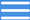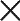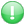MENUGet premium membership and access questions with answers, video lessons as well as revision papers.

# Grade 4 Mathematics Video Lessons on Fractions

"Welcome to our Grade 4 Mathematics video lessons on the topic of fractions. In this course, we'll be covering everything you need to know about fractions, including how to show and write them, writing numerators and denominators, different types of fractions, and how to convert improper fractions to mixed fractions and vice versa.
We'll start by explaining the basics of fractions, including what numerators and denominators are and how they're used.
Then, we'll move on to different types of fractions, such as proper and improper fractions.
You'll learn how to convert improper fractions to mixed fractions and mixed fractions to improper fractions.
By the end of this video, you'll have a solid understanding of fractions and be ready to tackle more advanced concepts. So, sit back, relax, and let's dive into the world of fractions!"

Lessons (13)